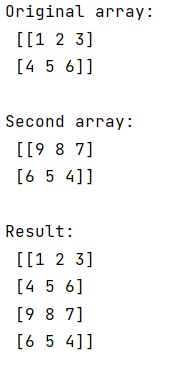# Concatenate two NumPy arrays vertically

Learn, how to concatenate two NumPy arrays vertically in Python?
Submitted by Pranit Sharma, on January 13, 2023

NumPy is an abbreviated form of Numerical Python. It is used for different types of scientific operations in python. Numpy is a vast library in python which is used for almost every kind of scientific or mathematical operation. It is itself an array which is a collection of various methods and functions for processing the arrays.

## NumPy - Concatenating two arrays vertically

Suppose that we are given a NumPy array and we want to concatenate this array with another NumPy array that too vertically.

For this purpose, we will use the numpy.concatenate() method. This method Joins a sequence of arrays along an existing axis. The arrays must have the same shape. The axis along which the arrays will be joined may be passed as an argument.

Let us understand with the help of an example,

## Python code to concatenate two NumPy arrays vertically

```# Import numpy
import numpy as np

# Creating a numpy array
arr = np.array([[1, 2, 3], [4, 5, 6]])

# Display original array
print("Original array:\n",arr,"\n")

# Creating another numpy array
arr2 = np.array([[9, 8, 7], [6, 5, 4]])

# Display second array
print("Second array:\n",arr2,"\n")

# concatenating both arrays
res = np.concatenate((arr,arr2),axis=0)

# Display Result
print("Result:\n",res)
```

Output:What's New (MCQs)

Top Interview Coding Problems/Challenges!

IncludeHelp's Blogs

Languages: » C » C++ » C++ STL » Java » Data Structure » C#.Net » Android » Kotlin » SQL
Web Technologies: » PHP » Python » JavaScript » CSS » Ajax » Node.js » Web programming/HTML
Solved programs: » C » C++ » DS » Java » C#
Aptitude que. & ans.: » C » C++ » Java » DBMS
Interview que. & ans.: » C » Embedded C » Java » SEO » HR
CS Subjects: » CS Basics » O.S. » Networks » DBMS » Embedded Systems » Cloud Computing
» Machine learning » CS Organizations » Linux » DOS
More: » Articles » Puzzles » News/Updates